﻿ Determination of Electrical Parameters of a CIGS-Based Solar Cell under the Influence of a KF Layer from Impedance SpectroscopyPublications are Open
Access in this journal
Article Versions
Export Article
Cite this article
• Normal Style
• MLA Style
• APA Style
• Chicago Style
Research Article
Open Access Peer-reviewed

### Determination of Electrical Parameters of a CIGS-Based Solar Cell under the Influence of a KF Layer from Impedance Spectroscopy

Djimba Niane , Ibrahima Wade, Ousmane Diagne, Mouhamadou M.Socé, Moustapha Dieng
American Journal of Energy Research. 2018, 6(2), 42-46. DOI: 10.12691/ajer-6-2-2
Received October 20, 2018; Revised November 25, 2018; Accepted December 09, 2018

### Abstract

The performance of CIGS-based solar cells is influenced by the phenomena occurring at the CIGS/CdS interface. In the present work, a study by the SCAPS-1D simulator of the influence of a KF layer incorporated into the CIGS/CdS interface is made with the impedance spectroscopy. The behavior of the dielectric response deduced from the Nyquist diagram shows an improvement of the shunt and serial resistance respectively when the KF is present. The Bode diagram shows a predominance of capacitive phenomena.

### 1. Introduction

Today, research in the photovoltaic field is directed towards the drastic reduction of production costs. This improvement in the quality/price ratio passes on the one hand by a need to find other materials that can substitute silicon and have good conversion efficiency. Indeed, there exist four under-sectors in the technology of the thin layers known as of second generation which presents the best efficiencies: amorphous silicon (10,2 %) 1, CdTe (21%) 1, le Cu (In, Ga) Se2 (22,6 %) 2 and Cu2ZnSnS4 (12,6 %) 3. Copper, indium and gallium diselenide type alloys, in particular Cu (In, Ga) Se2, are already available on the market and their record yield as mentioned above is between that of polycrystalline silicon and monocrystalline silicon. It is a very promising technology, nevertheless its industrial development necessarily involves the perfect mastery of all the properties of the different layers and all the parameters of the solar cell. Although studies have been made on the n-CdS/p-CIGS interface on the important role in carrier generation and recombination processes and the properties of the solar cell, its influences on device performance remain topics of discussions in the literature. Complex impedance spectroscopy is a well-known and powerful technique for studying the ac behavior dielectric materials 4. However, its use remains low in the case of heterojunction devices 5. The objective of this paper is to study by numerical simulation the influence of the thickness of the KF layer on the electrical properties of an n-ZnO/n-CdS/p-CIGS heterojunction using spectroscopy impedance. We give the variations of the shunt and series resistances and we propose an equivalent circuit.

### 2. Numerical Modeling

Modeling is widely used in the photovoltaic field to improve device performance. It allows to predict the effect of the variation of physical parameters on the performances of the cell and finally, to suggest the ways to follow to improve the performances of the device. There are several numerical simulation software developed by researchers in the field of thin films. Among these programs, we choose the SCAPS-1D software to do our study in this work 6, 7. The structure of the n-ZnO/n-CdS/p-CIGS heterojunction configured at the software, is shown in Figure 1. The KF layer incorporated into the CIGS/CdS interface, has a band gap of 1.5eV, its electronic affinity 4.14 eV and its dielectric constant 6.05 eV 8.

• Figure 1. Structure of a heterojunction solar cell n-ZnO/n-CdS/p-CIGS

The basic parameters of the four cell layers used in the simulation are shown in Table 1.

### 3. Results and Discussions

3.1. Defect Distribution

The calculation of the total distribution of the energy density of defects Nt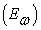is made by integrating it into each energy position occupied by the defects. His expression is given by 8:(1)

Where:

Ø Nt represents the density of defects,

Ø w the depletion width,

Ø q the elementary charge,

Ø C the capacity,

Ø Vbi is the built-in voltage,

Ø V the applied bias voltage,

Ø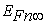a Fermi level energy,

Øthe temperature,

Ø And k the Boltzmann constant.

We represent the measurement of the distribution density of defects per energy according to the energy of the traps in Figure 2.

The slope and positive values of the density of queued distributions can be evaluated from the admittance data. The slope and positive values of tail-like density distributions can be evaluated from the admittance data. The profiles show a higher density of defects for the cell with KF. The distribution of Gaussian defects starts from 0.16 eV for both samples explained by an inaccuracy of the band profile but also the response associated with the modulation signal. A decrease in the defect density as a function of energy is noted for both cells. This decrease is much wider with the KF-free cell explained by the position of the Fermi level in the structure of band of CIGS layer, thus limiting the range in which defects in the lower band gap can be detected. Indeed, a state energy distribution requires a uniform distribution of defects in the space of both bands due to the fact that defects close to the Fermi level are detected. Thus, the variation of the angular frequency also involves a displacement of the position in the junction where the states are detected. Consequently, the defects closer to the interface are interpreted as deeper in energy.

3.2. Impedance Complex
3.2.1. Nyquist Diagram

CC the Nyquist diagram consists of representing the imaginary part of the impedance as a function of the real part of the impedance by varying the frequency. The purpose of a study of the diagram of Nyquist is to determine Shunt and series resistances respectively. The expression of the dynamic impedance is given by:(2)

With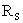andthe shunt and series resistances respectively and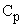the total capacity (sum between the capacity of diffusion and that of depletion).

• Figure 2. distribution density of defects per energy according to the energy of the traps in Figure 2
• Figure 3. Nyquist diagram for a cell with KF and without KF respectively
• Figure 4. the influence of the thickness of the KF layer on the series resistance

The theoretical values of the real and imaginary components of the impedance are expressed as follows: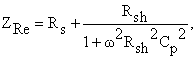(3)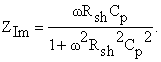(4)

Figure 3 shows the Nyquist diagram for a cell with KF and without KF respectively.

We notice that the Nyquist diagram gives semicircles explained by a predominance of a time constant. This time constant is given byThe semicircles obtained have a center of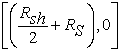and a diameter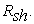We note an increase in the radius of curvature when the KF layer is present at the CIGS/CdS interface. For the low frequenciesthe real component of the impedance is equal to the sum of series resistance and shunt resistance whereas the imaginary part is zero. For high frequencies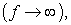the real part of the impedance is equal to the series resistance and the imaginary part of the impedance tends to zero. The series and shunt resistance values found are summarized in the table below.

It appears in this table an increase in shunt resistance but also in the series resistance when the KF layer is present at the cell. We vary the thickness of the KF layer at the interface to see its effect on the series and shunt resistances respectively.

Figure 4 shows the influence of the thickness of the KF layer on the series resistance of the CIGS-based solar cell.

We varied the thickness of the KF layer in steps of 5nm. The plot shows an increase in the series resistance as a function of the thickness of the KF layer to a peak corresponding to the thickness 25 nm. Then we note a decrease to a depth corresponding to the thickness 40nm and follows a further increase. Considering that the series resistance characterizes the ohmic losses due to the Joule effect and the losses through the grids of collection and the ohmic bad contacts. Therefore, the increase of the series resistance can be explained by the resistivity of the CIGS layer for KF content values between 0 and 25nm. While the decrease in series resistance is related to the diffusion of potassium in the CIGS layer that improves the concentration of carriers. Moreover, we notice low values of series resistance what more proves the effects beneficial of potassium in the fabrication t of this type of solar cells.

Figure 5 shows the evolution of the shunt resistance as a function of the thickness of the KF layer.

We observe in the Figure 5 that the shunt resistance increases as a function of the thickness of the KF layer. Since the shunt resistor characterizes the recombination losses due to the thicknesses of the N, P regions and the space charge zone. It has a determining role in the optimization of solar cells because it is linked by the structural defects existing within the material (dislocations) and also in the elaboration process. Thus, the increase of the shunt resistance is caused by the decrease of the leakage current which consequently leads to an increase in the output current of the photovoltaic cell. In addition, increasing this resistance allows the cell to absorb more current, since the current flowing through the heterojunction improves considerably.

3.2.2. Bode Diagram

The Bode diagram is used to represent the harmonic response of a dynamic system. It makes it possible to trace the amplitude and the phase φ of the impedance as a function of the pulsation ω of the sinusoidal excitation. So it has two parts, the amplitude diagram and the phase diagram.

The impedance module diagram for the two samples is shown in Figure 6.

• Figure 5. evolution of the shunt resistance as a function of the thickness of the KF layer.
• Figure 6. impedance module diagram as a function of the pulsation ω for a cell with KF and without KF respectively
• Figure 7. phase of the impedance as a function of the pulsation

It is observed that the amplitude of the impedance is more important with the cell which presents a layer of KF than with the cell which is deprived of KF. It also results in Figure 6 that the impedance modulus is constant for frequencies below the cutoff frequency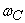and decreases asymptotically when the frequencies are greater than. The cutoff frequency is obtained by intersecting the extensions of each of the two linear parts of the curve. The Table 3 below gives the values of the cutoff pulsation obtained for the two samples.

We proceed to the representation of the phase of the impedance as a function of the pulsation shown in Figure 7.

We note that the phase of the impedance decreases as a function of the pulsation. This phase also decreases with the presence of KF at the cell as shown by the zoom inside Figure 7. In addition, we note a negative phase that shows the predominance of capacitive phenomena. Therefore we can propose an impedance equivalent circuit consisting of a capacitance, a shunt (parallel) resistance and a series resistance.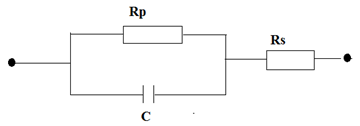### 4. Conclusion

We studied by simulation in this work a heterojunction n-ZnO/n-CdS/p-CIGS under the influence of the thickness of a KF layer incorporated in the CIGS/CdS interface by using impedance spectroscopy. The observation made from the Nyquist diagrams shows semicircles. In addition, we noted that the variation of the series and shunt resistances respectively is not homogeneous with the increase of the quantity of KF. However, the series resistance remains low while the shunt resistance increases with the KF. The Bode diagram showed the predominance of capacitive phenomena. This behavior implies that the observed dielectric response can be described by an equivalent circuit consisting of a shunt resistance Rp and a capacitor C connected in series with a series resistances Rs.

### Reference

  M. A. Green, K. Emery, Y. Hishikawa, W. Warta, and E. D. Dunlop, “Solarcell efficiency tables (Version 45)”, Progress in Photovoltaics: Research and Applications, vol. 23, no. 1, pp. 1-9, 2015. In article View Article  Philip Jackson , Roland Wuerz, Dimitrios Hariskos, Erwin Lotter, Wolfram Witte, and Michael Powalla, “Effects of heavy alkali elements in Cu(In,Ga)Se2solar cells with efficiencies up to 22.6%”, Phys. Status Solidi RRL 10, No. 8, pp: 583-586, 2016. In article View Article  M. A. Green, K. Emery, Y. Hishikawa, W. Warta, and E. D. Dunlop, “Solarcell efficiency tables (version 44),” Progress in Photovoltaics: Research andApplications, vol. 22, no. 7, pp: 701-710, 2014. In article  J.R. Mac Donald, W.B. Johnson, Impedance Spectroscopy, Wiley, New York, 1987 In article  R.A. Kumar, M.S. Suresh and J. Nagaraju, “Measurement and comparison of AC parameters of silicon (BSR and BSFR) and gallium arsenide (GaAs/Ge) solar cells used in space applications” Sol. Energy Mat. And Sol. Cells., 60, (2000), 155. In article View Article  M. Burgelman, P. Nollet, S. Degrave, “Modelling polycrystalline semiconductor solar cells”, Thin Solid Films 361/362. (2000). pp. 527-532. In article View Article  M. Burgelman, K. Decock, S. Khelifi and A. Abass, “Advanced electrical simulation of thin film solar cells”, Thin Solid Films, 535. (2013). 296-30. In article View Article  N.F. Mott & R.W. Gurney, “Electronic processes in Ionic crystals”, Dover, New York 1964. pp: 274. In article  T. Walter, et al., “Determination of defect distributions from admittance measurements and application to Cu (In, Ga) Se2 based heterojunctions,” Journal of Applied Physics, Vol 80, (October 1996). pp. 4411-4420. In article View Article

Published with license by Science and Education Publishing, Copyright © 2018 Djimba Niane, Ibrahima Wade, Ousmane Diagne, Mouhamadou M.Socé and Moustapha DiengThis work is licensed under a Creative Commons Attribution 4.0 International License. To view a copy of this license, visit http://creativecommons.org/licenses/by/4.0/

### Cite this article:

##### Normal Style
Djimba Niane, Ibrahima Wade, Ousmane Diagne, Mouhamadou M.Socé, Moustapha Dieng. Determination of Electrical Parameters of a CIGS-Based Solar Cell under the Influence of a KF Layer from Impedance Spectroscopy. American Journal of Energy Research. Vol. 6, No. 2, 2018, pp 42-46. http://pubs.sciepub.com/ajer/6/2/2
##### MLA Style
Niane, Djimba, et al. "Determination of Electrical Parameters of a CIGS-Based Solar Cell under the Influence of a KF Layer from Impedance Spectroscopy." American Journal of Energy Research 6.2 (2018): 42-46.
##### APA Style
Niane, D. , Wade, I. , Diagne, O. , M.Socé, M. , & Dieng, M. (2018). Determination of Electrical Parameters of a CIGS-Based Solar Cell under the Influence of a KF Layer from Impedance Spectroscopy. American Journal of Energy Research, 6(2), 42-46.
##### Chicago Style
Niane, Djimba, Ibrahima Wade, Ousmane Diagne, Mouhamadou M.Socé, and Moustapha Dieng. "Determination of Electrical Parameters of a CIGS-Based Solar Cell under the Influence of a KF Layer from Impedance Spectroscopy." American Journal of Energy Research 6, no. 2 (2018): 42-46.
Share